## The Gradient and Directional Derivative

The gradient of a function w=f(x,y,z) is the vector function: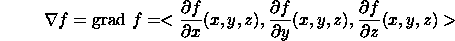For a function of two variables z=f(x,y), the gradient is the two-dimensional vector <f_x(x,y),f_y(x,y)>. This definition generalizes in a natural way to functions of more than three variables.

Examples

For the function z=f(x,y)=4x^2+y^2. The gradient is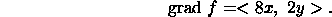For the function w=g(x,y,z)=exp(xyz)+sin(xy), the gradient is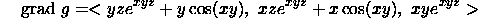Geometric Description of the Gradient Vector

There is a nice way to describe the gradient geometrically. Consider z=f(x,y)=4x^2+y^2. The surface defined by this function is an elliptical paraboloid. This is a bowl-shaped surface. The bottom of the bowl lies at the origin. The figure below shows the level curves, defined by f(x,y)=c, of the surface. The level curves are the ellipses 4x^2+y^2=c.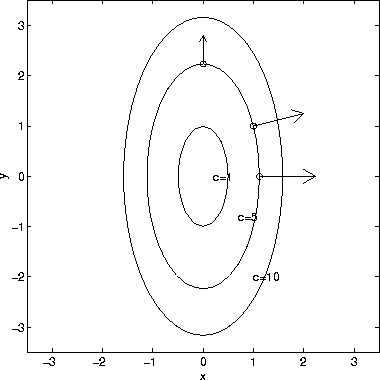The gradient vector <8x,2y> is plotted at the 3 points (sqrt(1.25),0), (1,1), (0,sqrt(5)). As the plot shows, the gradient vector at (x,y) is normal to the level curve through (x,y). As we will see below, the gradient vector points in the direction of greatest rate of increase of f(x,y)

In three dimensions the level curves are level surfaces. Again, the gradient vector at (x,y,z) is normal to level surface through (x,y,z).

Directional Derivatives

For a function z=f(x,y), the partial derivative with respect to x gives the rate of change of f in the x direction and the partial derivative with respect to y gives the rate of change of f in the y direction. How do we compute the rate of change of f in an arbitrary direction?

The rate of change of a function of several variables in the direction u is called the directional derivative in the direction u. Here u is assumed to be a unit vector. Assuming w=f(x,y,z) and u=<u_1,u_2,u_3>, we have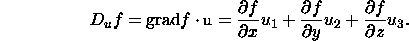Hence, the directional derivative is the dot product of the gradient and the vector u. Note that if u is a unit vector in the x direction, u=<1,0,0>, then the directional derivative is simply the partial derivative with respect to x. For a general direction, the directional derivative is a combination of the all three partial derivatives.

Example

What is the directional derivative in the direction <1,2> of the function z=f(x,y)=4x^2+y^2 at the point x=1 and y=1. The gradient is <8x,2y>, which is <8,2> at the point x=1 and y=1. The direction u is <2,1>. Converting this to a unit vector, we have <2,1>/sqrt(5). Hence,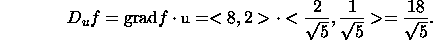Directions of Greatest Increase and Decrease

The directional derivative can also be written: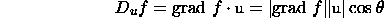where theta is the angle between the gradient vector and u. The directional derivative takes on its greatest positive value if theta=0. Hence, the direction of greatest increase of f is the same direction as the gradient vector. The directional derivative takes on its greatest negative value if theta=pi (or 180 degrees). Hence, the direction of greatest decrease of f is the direction opposite to the gradient vector.